### Home > PC > Chapter 8 > Lesson 8.1.2 > Problem8-24

8-24.
1. Evaluate the following limits. (Note the negative sign in parts (a), (b), and (c).) Homework Help ✎

1.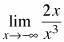2.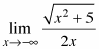3.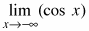4.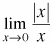5.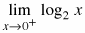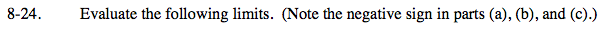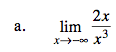The dominant term is in the denominator.
As x→∞, the fraction gets closer to 0.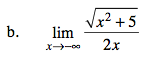The dominant term is |x| in the numerator (square root of x2 is |x|) and x in the denominator, so the answer is the ratio of the coefficients.

$\text{i.e. }\frac{1}{-2}$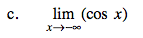The cosine function oscillates between 1 and −1 forever. Since a limit must approach a single height, the limit does not exist.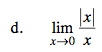Is the graph approaching the SAME height from both sides?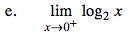What height is the graph approaching as x→0+?